Uses a non-parametric approach to reconstruct cases by date of infection from reported cases. It uses either a generative Rt model or non-parametric back calculation to estimate underlying latent infections and then maps these infections to observed cases via uncertain reporting delays and a flexible observation model. See the examples and function arguments for the details of all options. The default settings may not be sufficient for your use case so the number of warmup samples (stan_args = list(warmup)) may need to be increased as may the overall number of samples. Follow the links provided by any warnings messages to diagnose issues with the MCMC fit. It is recommended to explore several of the Rt estimation approaches supported as not all of them may be suited to users own use cases. See here for an example of using estimate_infections within the epinow wrapper to estimate Rt for Covid-19 in a country from the ECDC data source.

estimate_infections(
reported_cases,
generation_time,
delays = delay_opts(),
truncation = trunc_opts(),
rt = rt_opts(),
backcalc = backcalc_opts(),
gp = gp_opts(),
obs = obs_opts(),
stan = stan_opts(),
horizon = 7,
CrIs = c(0.2, 0.5, 0.9),
zero_threshold = 50,
id = "estimate_infections",
verbose = interactive()
)

## Arguments

reported_cases

A data frame of confirmed cases (confirm) by date (date). confirm must be integer and date must be in date format.

generation_time

A list containing the mean, standard deviation of the mean (mean_sd), standard deviation (sd), standard deviation of the standard deviation and the maximum allowed value for the generation time (assuming a gamma distribution).

delays

A call to delay_opts() defining delay distributions and options. See the documentation of delay_opts() and the examples below for details.

truncationA list of options as generated by trunc_opts() defining the truncation of observed data. Defaults to trunc_opts(). See estimate_truncation() for an approach to estimating truncation from data.

rt

A list of options as generated by rt_opts() defining Rt estimation. Defaults to rt_opts(). Set to NULL to switch to using back calculation rather than generating infections using Rt.

backcalc

A list of options as generated by backcalc_opts() to define the back calculation. Defaults to backcalc_opts().

gp

A list of options as generated by gp_opts() to define the Gaussian process. Defaults to gp_opts().Set to NULL to disable the Gaussian process.

obs

A list of options as generated by obs_opts() defining the observation model. Defaults to obs_opts().

stan

A list of stan options as generated by stan_opts(). Defaults to stan_opts(). Can be used to override data, init, and verbose settings if desired.

horizon

Numeric, defaults to 7. Number of days into the future to forecast.

CrIs

Numeric vector of credible intervals to calculate.

zero_thresholdNumeric defaults to 50. Indicates if detected zero cases are meaningful by using a threshold of 50 cases on average over the last 7 days. If the average is above this threshold then the zero is replaced with the backwards looking rolling average. If set to infinity then no changes are made.

id

A character string used to assign logging information on error. Used by regional_epinow to assign errors to regions. Alter the default to run with error catching.

verbose

Logical, defaults to TRUE when used interactively and otherwise FALSE. Should verbose debug progress messages be printed. Corresponds to the "DEBUG" level from futile.logger. See setup_logging for more detailed logging options.

epinow regional_epinow forecast_infections simulate_infections

## Examples

# \donttest{
# set number of cores to use
options(mc.cores = ifelse(interactive(), 4, 1))
# get example case counts
reported_cases <- example_confirmed[1:60]

# set up example generation time
generation_time <- get_generation_time(disease = "SARS-CoV-2", source = "ganyani")
# set delays between infection and case report
incubation_period <- get_incubation_period(disease = "SARS-CoV-2", source = "lauer")
reporting_delay <- list(
mean = convert_to_logmean(2, 1), mean_sd = 0.1,
sd = convert_to_logsd(2, 1), sd_sd = 0.1, max = 10
)

# default setting
# here we assume that the observed data is truncated by the same delay as
def <- estimate_infections(reported_cases,
generation_time = generation_time,
delays = delay_opts(incubation_period, reporting_delay),
rt = rt_opts(prior = list(mean = 2, sd = 0.1)),
stan = stan_opts(control = list(adapt_delta = 0.95))
)
# real time estimates
summary(def)
#>                                  measure                estimate
#> 1: New confirmed cases by infection date     2223 (1165 -- 4101)
#> 2:        Expected change in daily cases       Likely decreasing
#> 3:            Effective reproduction no.      0.86 (0.61 -- 1.1)
#> 4:                        Rate of growth -0.038 (-0.12 -- 0.039)
#> 5:          Doubling/halving time (days)          -18 (18 -- -6)
# summary plot
plot(def)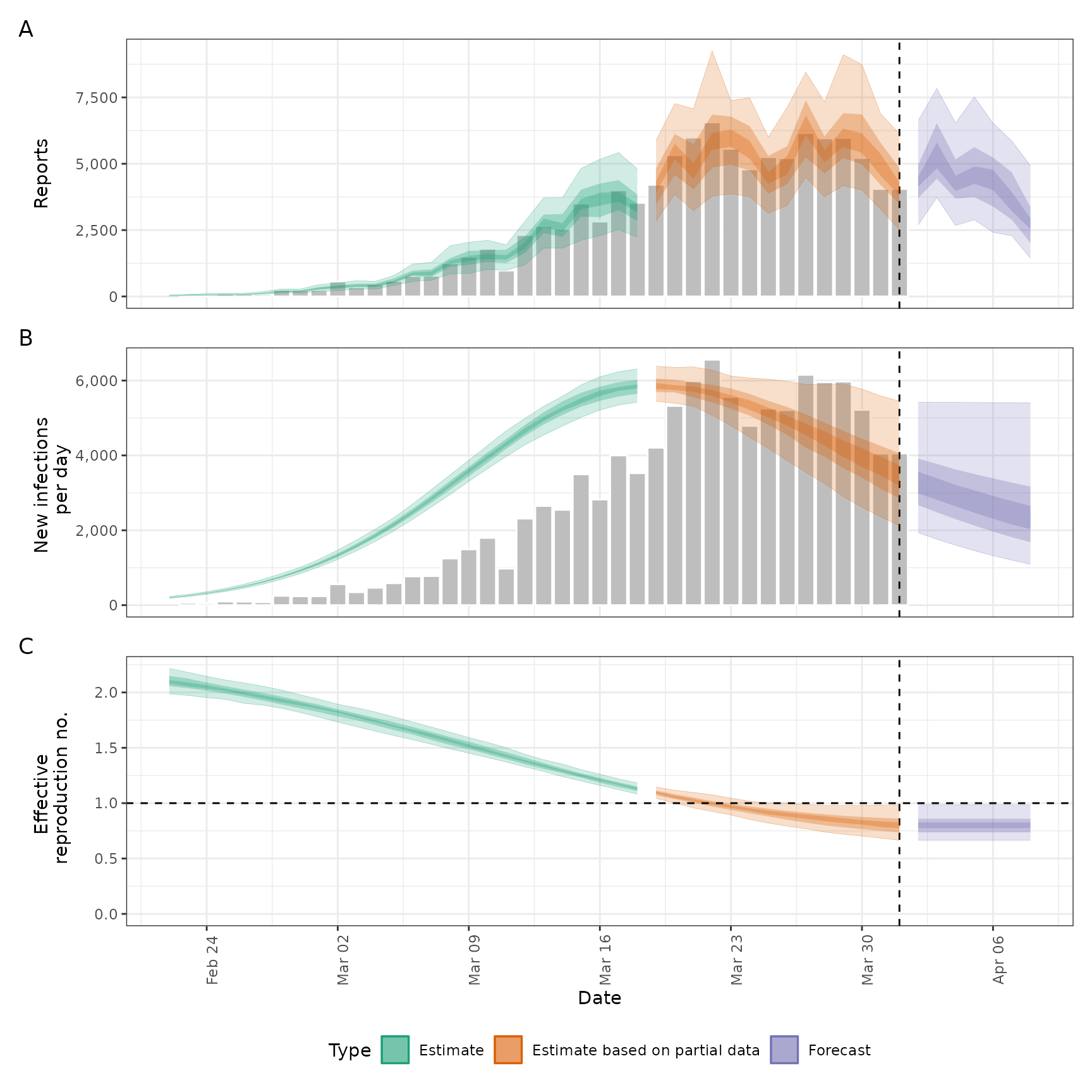# decreasing the accuracy of the approximate Gaussian to speed up computation.
# These settings are an area of active research. See ?gp_opts for details.
agp <- estimate_infections(reported_cases,
generation_time = generation_time,
delays = delay_opts(incubation_period, reporting_delay),
rt = rt_opts(prior = list(mean = 2, sd = 0.1)),
gp = gp_opts(ls_min = 10, basis_prop = 0.1),
stan = stan_opts(control = list(adapt_delta = 0.95))
)
summary(agp)
#>                                  measure                estimate
#> 1: New confirmed cases by infection date     2314 (1238 -- 4170)
#> 2:        Expected change in daily cases       Likely decreasing
#> 3:            Effective reproduction no.      0.88 (0.63 -- 1.2)
#> 4:                        Rate of growth -0.033 (-0.11 -- 0.044)
#> 5:          Doubling/halving time (days)        -21 (16 -- -6.5)
plot(agp)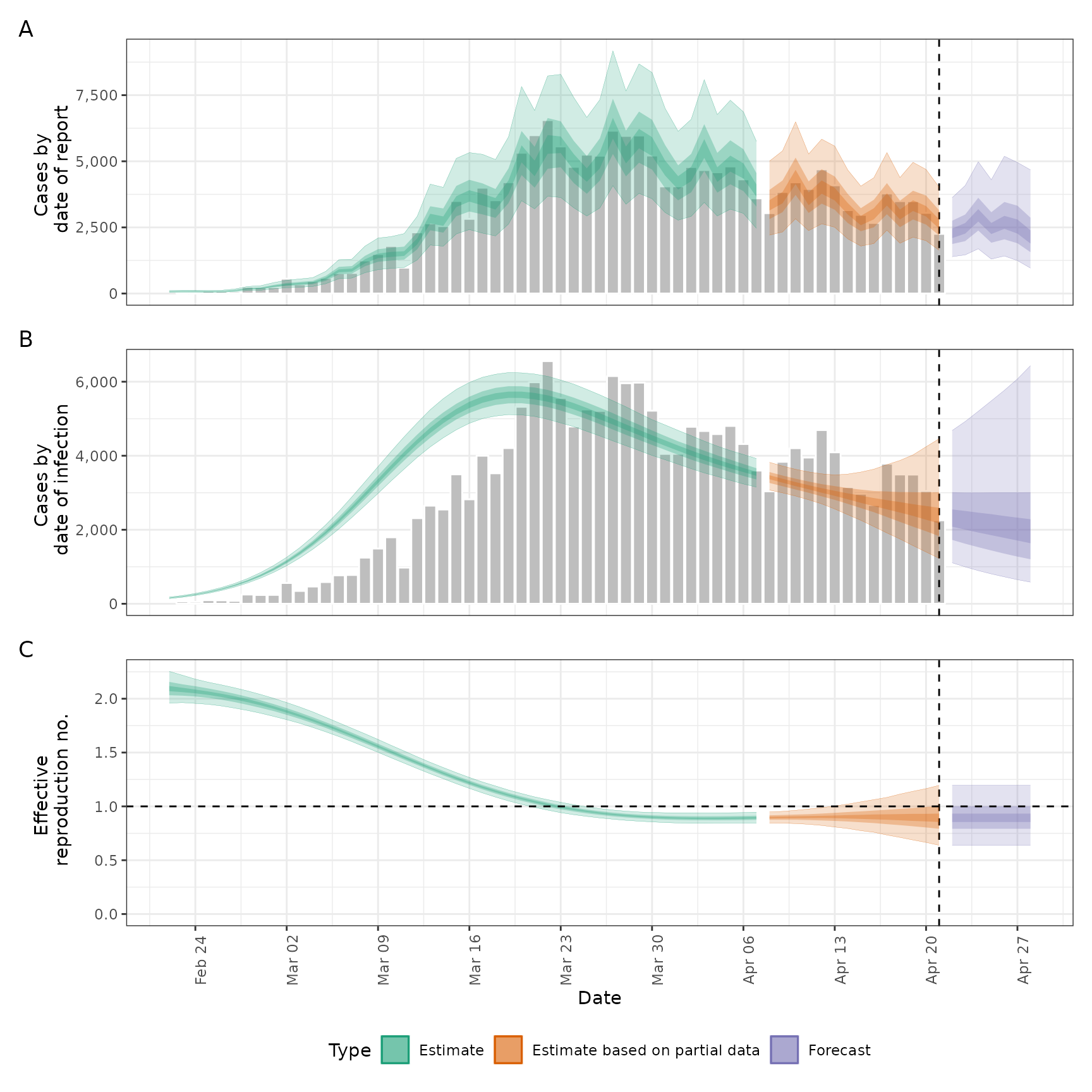# Adjusting for future susceptible depletion
dep <- estimate_infections(reported_cases,
generation_time = generation_time,
delays = delay_opts(incubation_period, reporting_delay),
rt = rt_opts(
prior = list(mean = 2, sd = 0.1),
pop = 1000000, future = "latest"
),
gp = gp_opts(ls_min = 10, basis_prop = 0.1), horizon = 21,
stan = stan_opts(control = list(adapt_delta = 0.95))
)
plot(dep)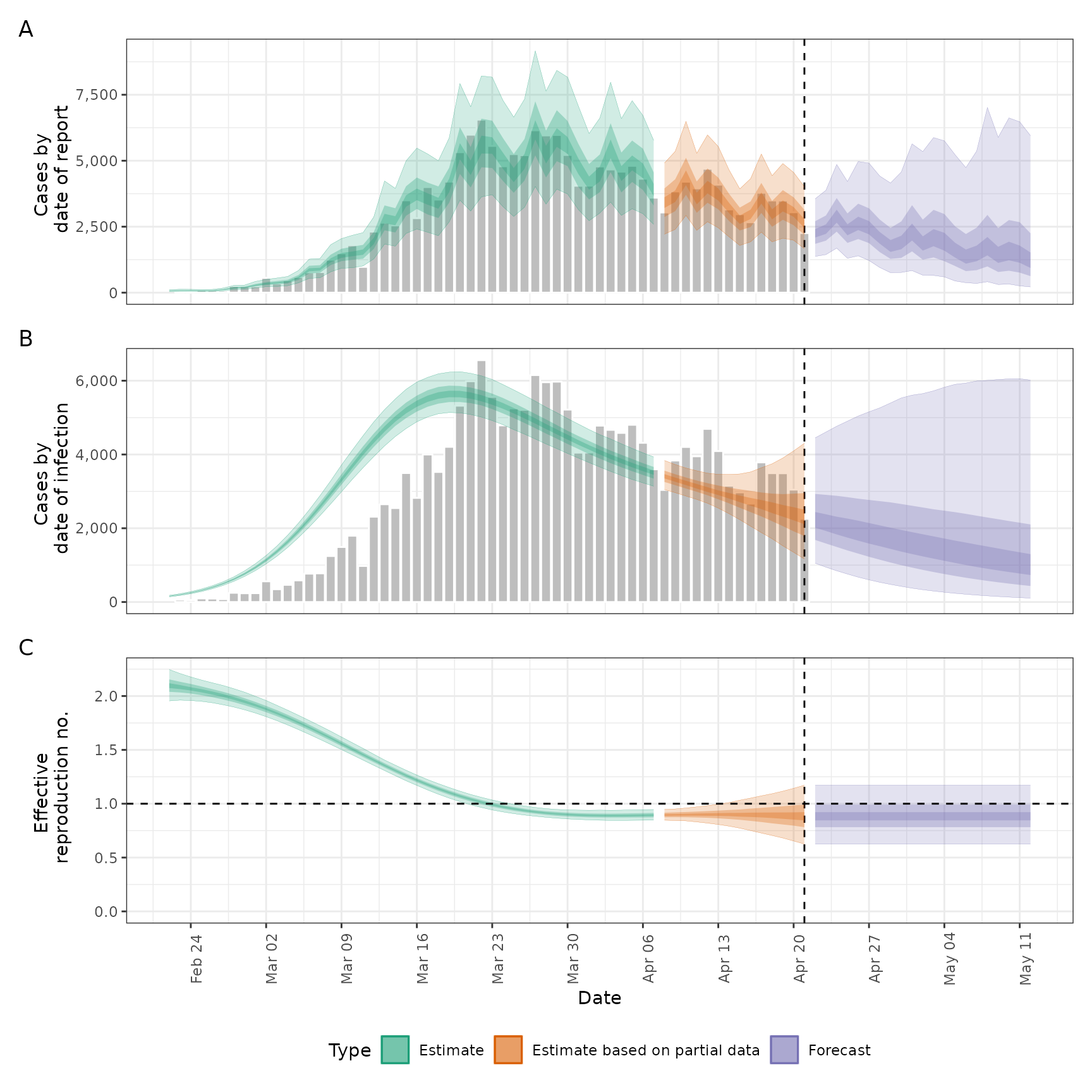# Adjusting for truncation of the most recent data
# See estimate_truncation for an approach to estimating this from data
trunc_dist <- list(
mean = convert_to_logmean(0.5, 0.5), mean_sd = 0.1,
sd = convert_to_logsd(0.5, 0.5), sd_sd = 0.1,
max = 3
)
trunc <- estimate_infections(reported_cases,
generation_time = generation_time,
delays = delay_opts(incubation_period, reporting_delay),
truncation = trunc_opts(trunc_dist),
rt = rt_opts(prior = list(mean = 2, sd = 0.1)),
gp = gp_opts(ls_min = 10, basis_prop = 0.1),
stan = stan_opts(control = list(adapt_delta = 0.95))
)
plot(trunc)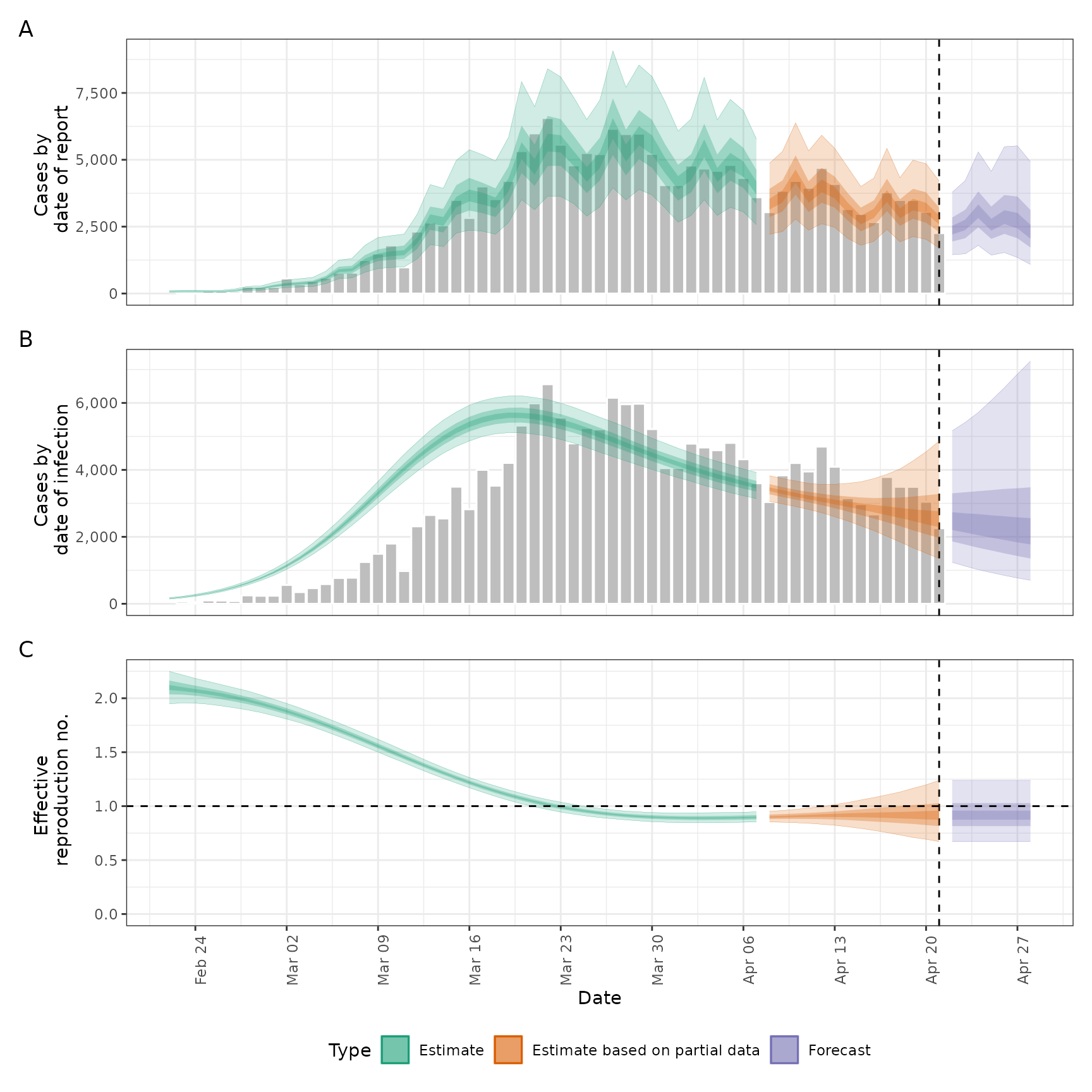# using back calculation (combined here with under reporting)
# this model is in the order of 10 ~ 100 faster than the gaussian process method
# it is likely robust for retrospective Rt but less reliable for real time estimates
# the width of the prior window controls the reliance on observed data and can be
# optionally switched off using backcalc_opts(prior = "none"), see ?backcalc_opts for
# other options
backcalc <- estimate_infections(reported_cases,
generation_time = generation_time,
delays = delay_opts(incubation_period, reporting_delay),
rt = NULL, backcalc = backcalc_opts(),
obs = obs_opts(scale = list(mean = 0.4, sd = 0.05)),
horizon = 0
)
plot(backcalc)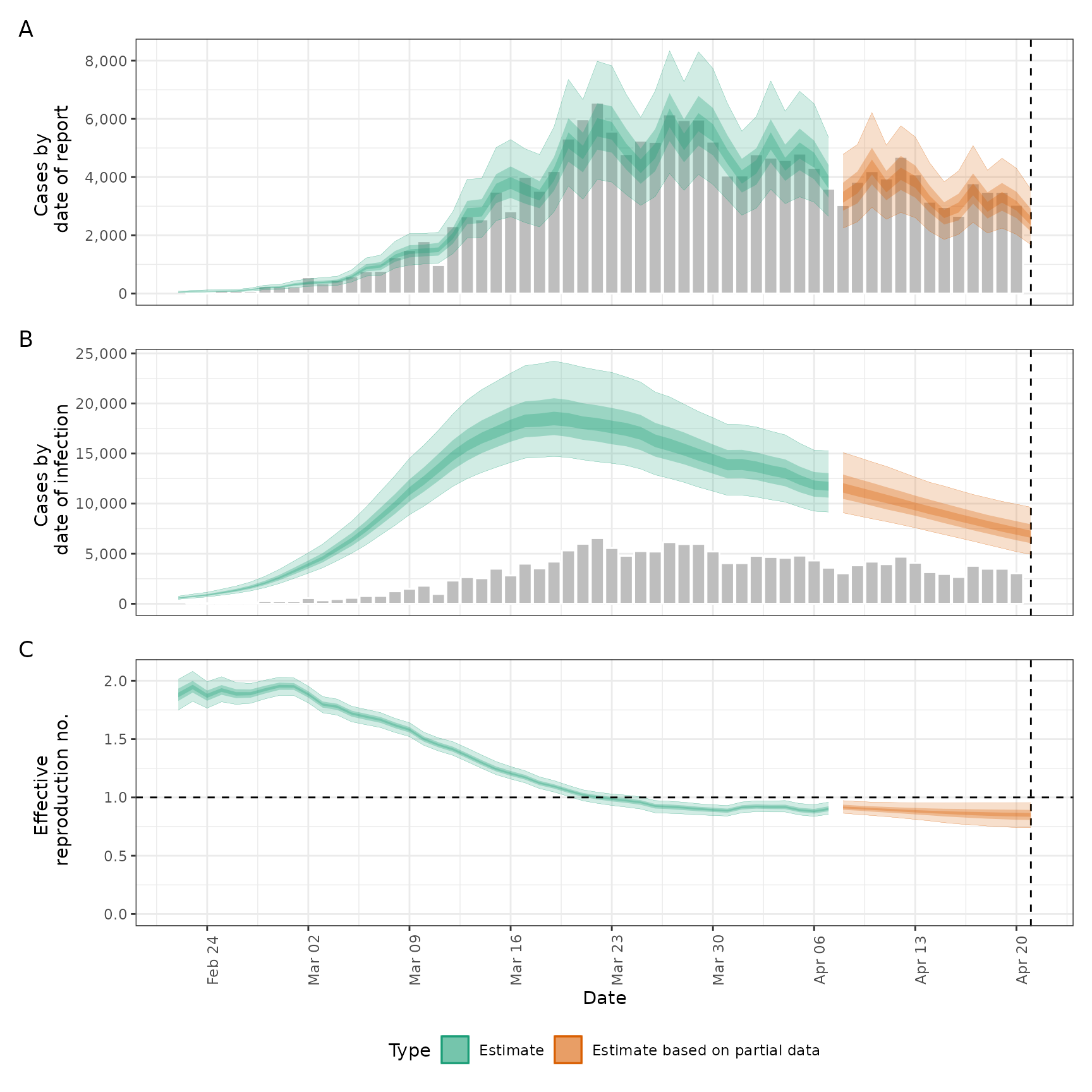# Rt projected into the future using the Gaussian process
project_rt <- estimate_infections(reported_cases,
generation_time = generation_time,
delays = delay_opts(incubation_period, reporting_delay),
rt = rt_opts(
prior = list(mean = 2, sd = 0.1),
future = "project"
)
)
plot(project_rt)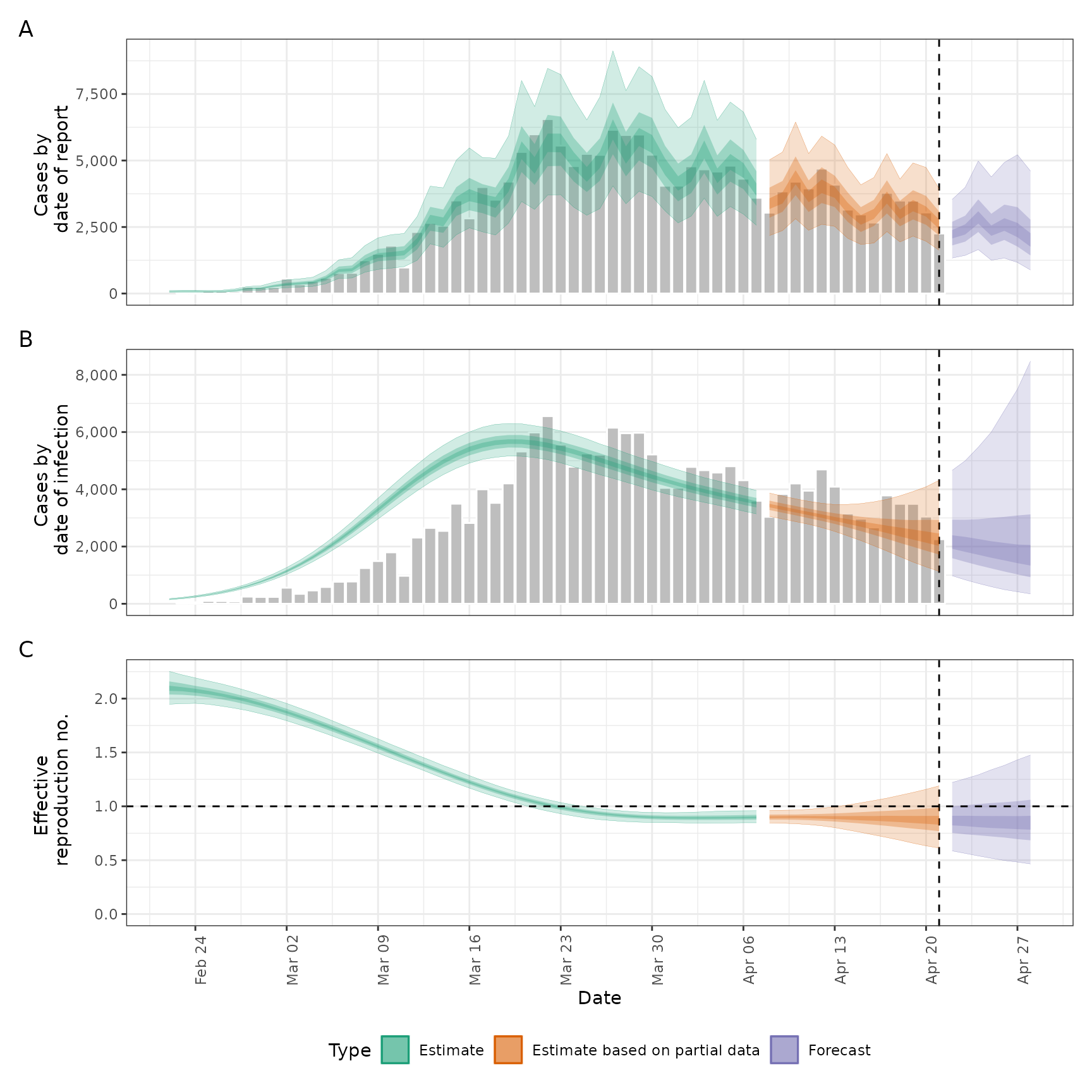# default settings on a later snapshot of data
snapshot_cases <- example_confirmed[80:130]
snapshot <- estimate_infections(snapshot_cases,
generation_time = generation_time,
delays = delay_opts(incubation_period, reporting_delay),
rt = rt_opts(prior = list(mean = 1, sd = 0.1))
)
plot(snapshot)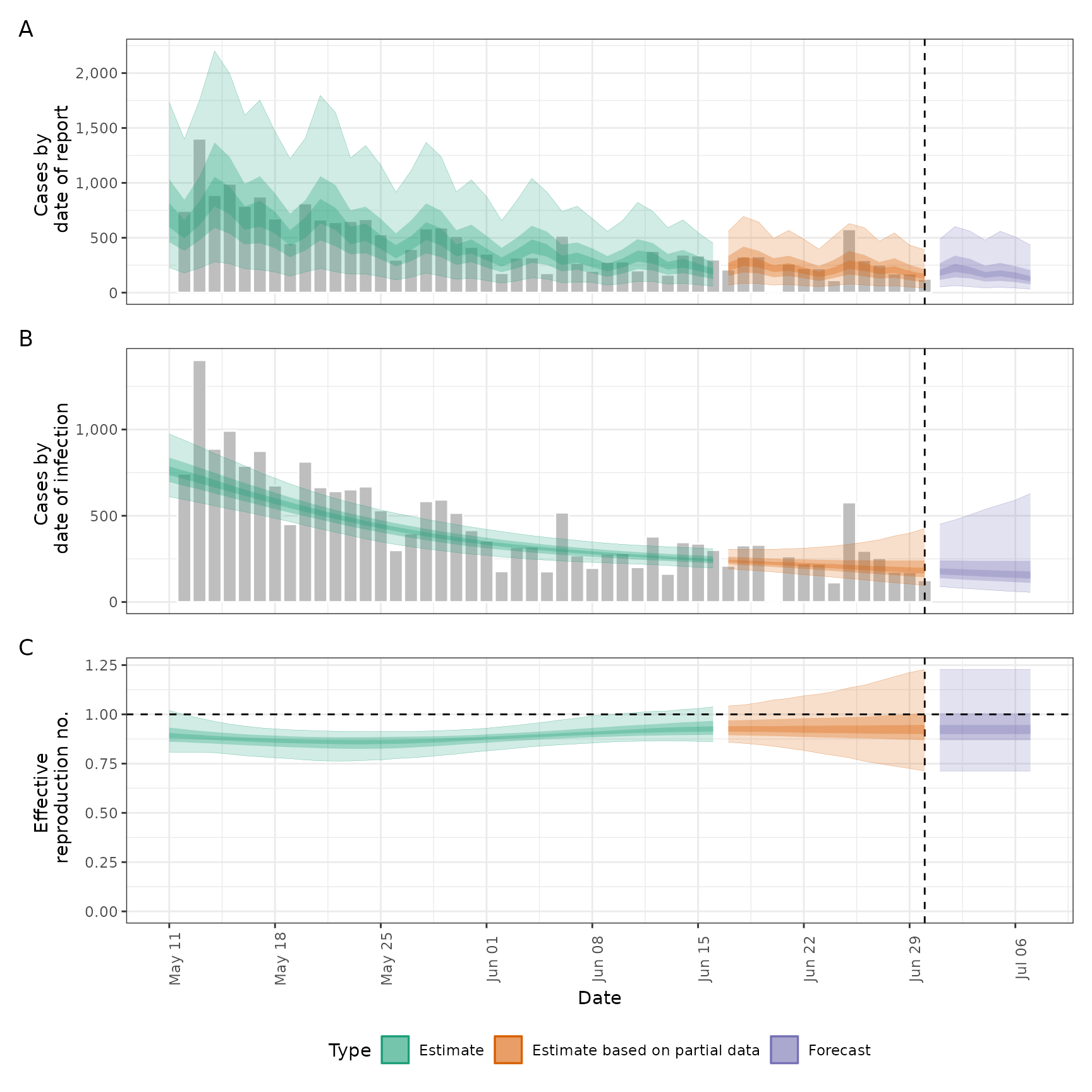# stationary Rt assumption (likely to provide biased real-time estimates)
stat <- estimate_infections(reported_cases,
generation_time = generation_time,
delays = delay_opts(incubation_period, reporting_delay),
rt = rt_opts(prior = list(mean = 2, sd = 0.1), gp_on = "R0")
)
plot(stat)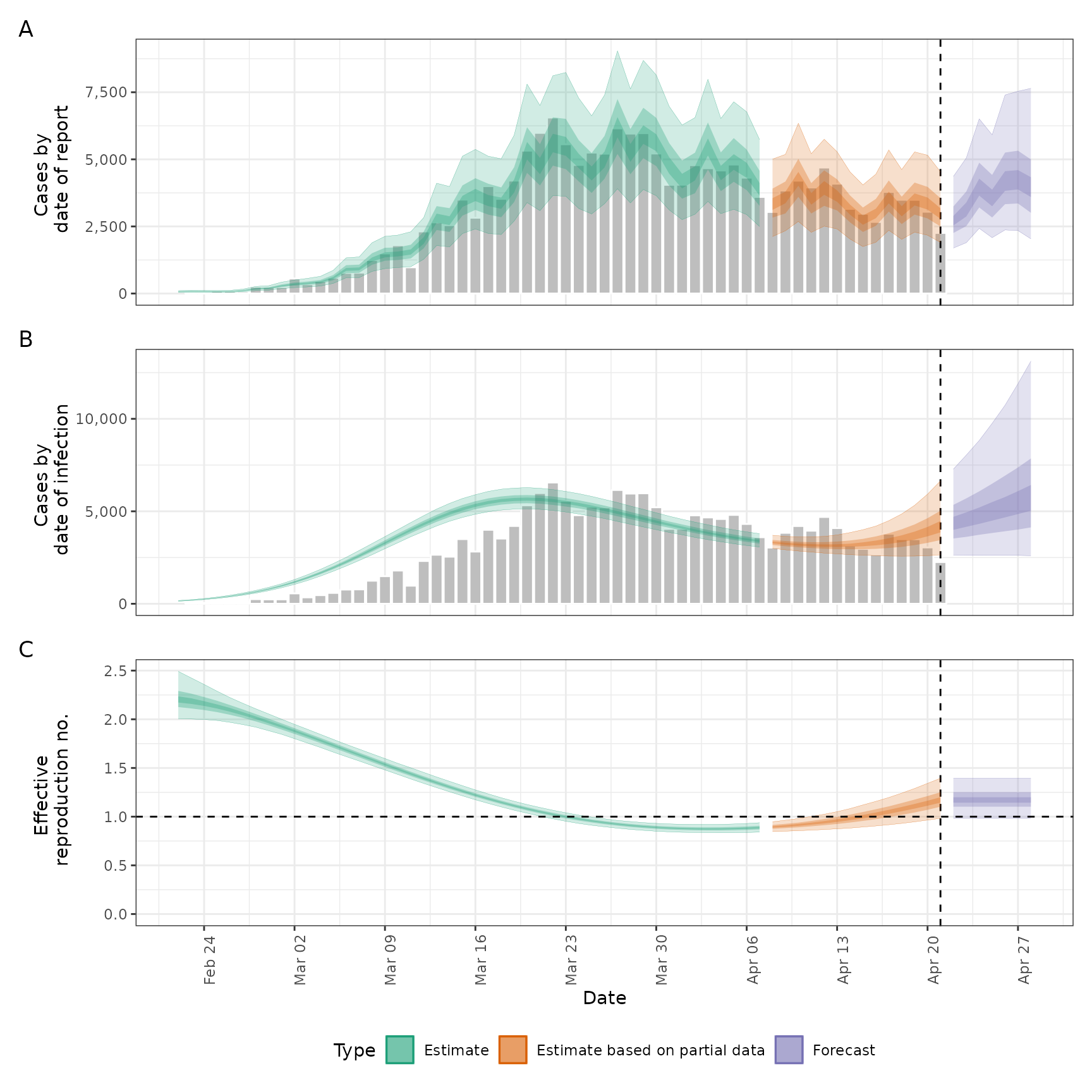# no gaussian process (i.e fixed Rt assuming no breakpoints)
fixed <- estimate_infections(reported_cases,
generation_time = generation_time,
delays = delay_opts(incubation_period, reporting_delay),
gp = NULL
)
plot(fixed)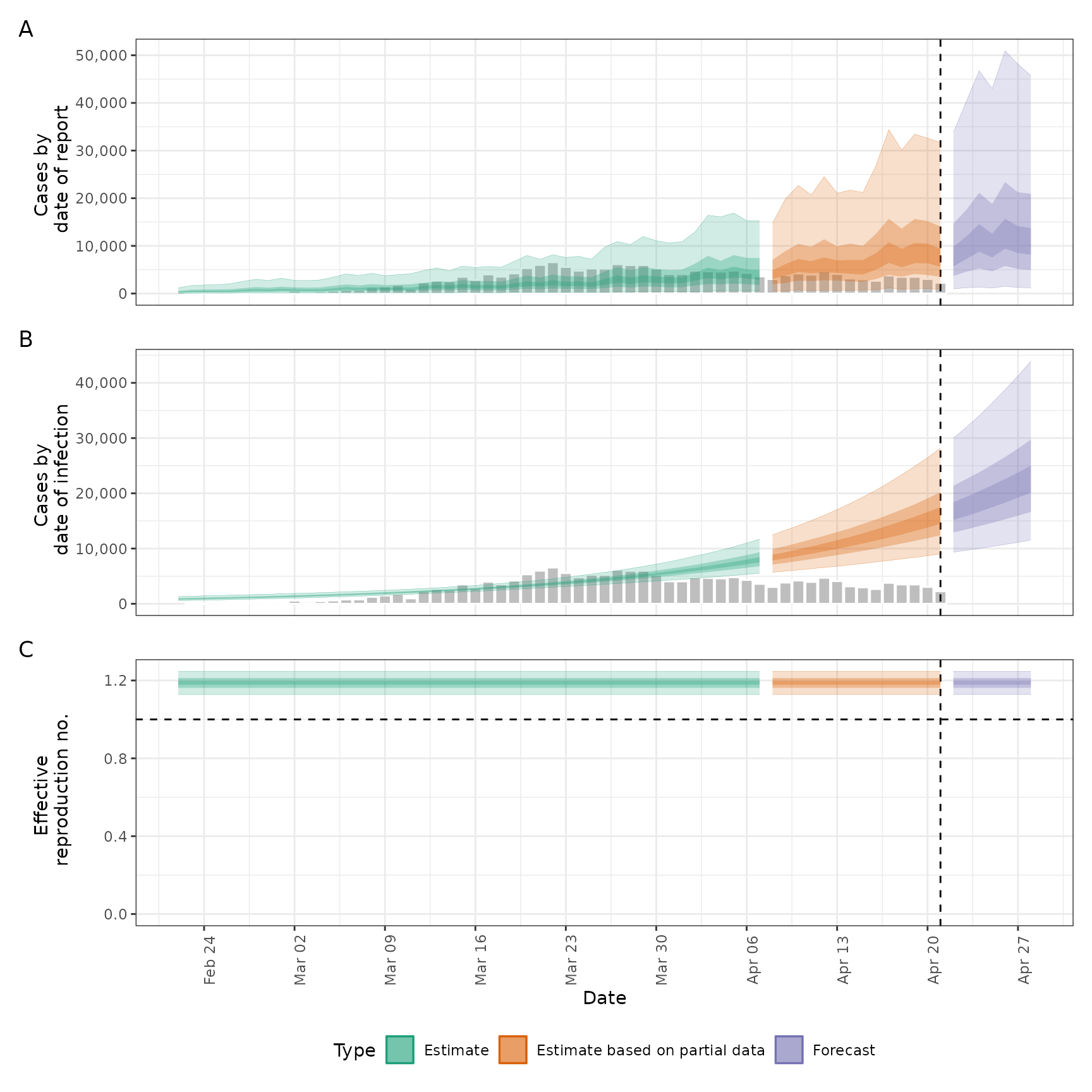# no delays
no_delay <- estimate_infections(reported_cases, generation_time = generation_time)
plot(no_delay)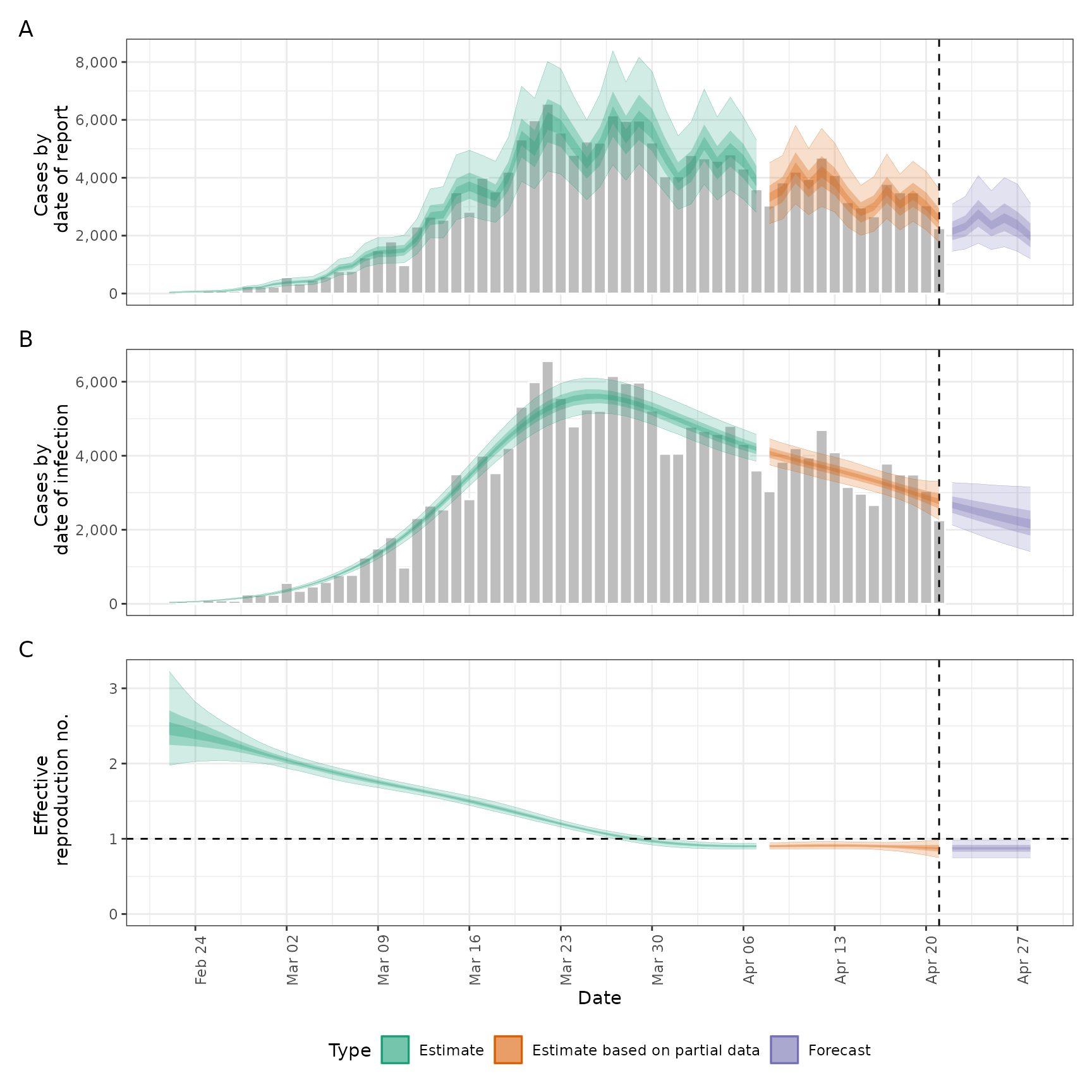# break point but otherwise static Rt
bp_cases <- data.table::copy(reported_cases)
bp_cases <- bp_cases[, breakpoint := ifelse(date == as.Date("2020-03-16"), 1, 0)]
bkp <- estimate_infections(bp_cases,
generation_time = generation_time,
delays = delay_opts(incubation_period, reporting_delay),
rt = rt_opts(prior = list(mean = 2, sd = 0.1)),
gp = NULL
)
# break point effect
summary(bkp, type = "parameters", params = "breakpoints")
#>    date    variable strat type     median       mean         sd   lower_90
#> 1: <NA> breakpoints     1 <NA> -0.6601589 -0.6605438 0.02735112 -0.7053991
#>      lower_50   lower_20   upper_20   upper_50   upper_90
#> 1: -0.6787856 -0.6670959 -0.6536662 -0.6423823 -0.6122912
plot(bkp)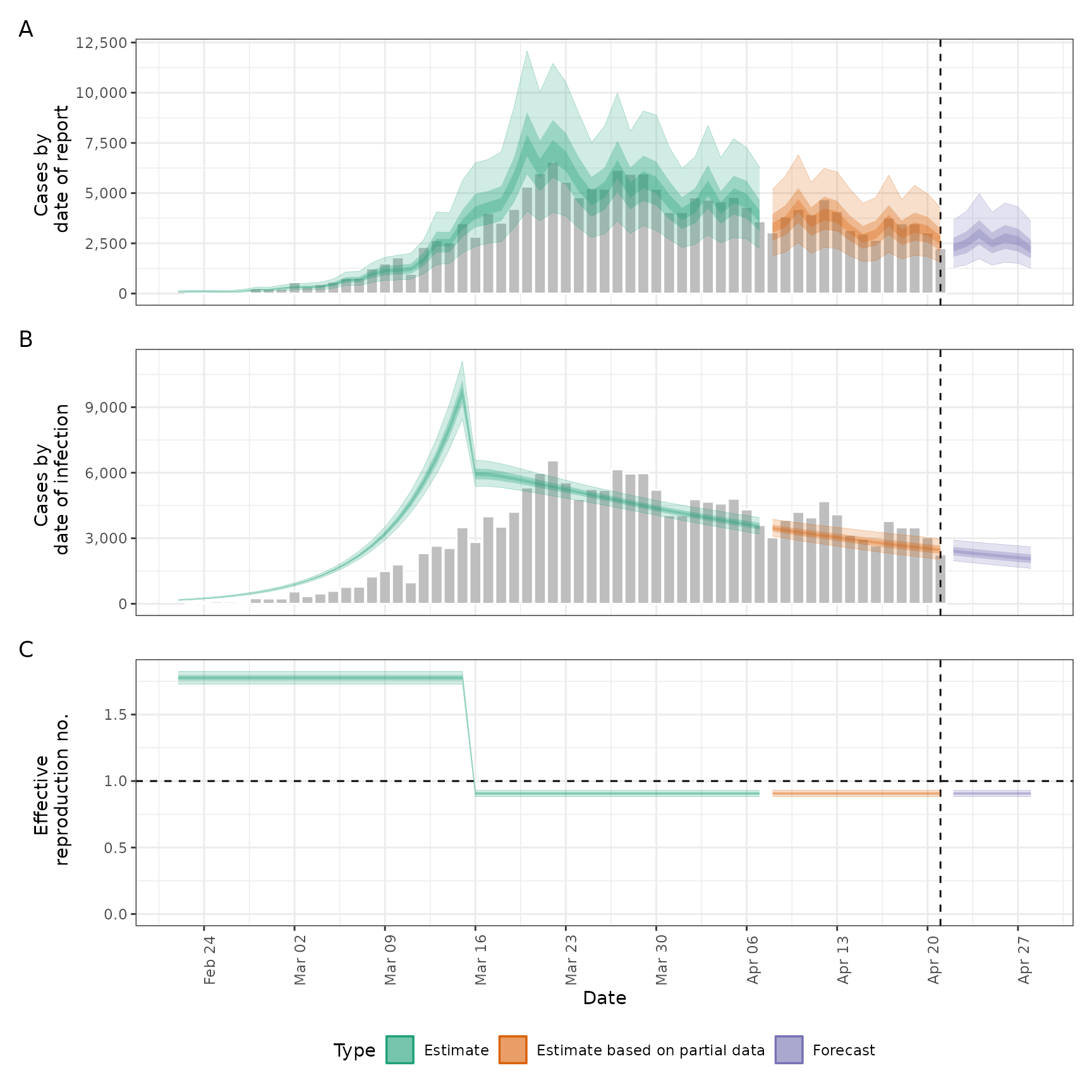# weekly random walk
rw <- estimate_infections(reported_cases,
generation_time = generation_time,
delays = delay_opts(incubation_period, reporting_delay),
rt = rt_opts(prior = list(mean = 2, sd = 0.1), rw = 7),
gp = NULL
)

# random walk effects
summary(rw, type = "parameters", params = "breakpoints")
#>    date    variable strat type      median        mean         sd   lower_90
#> 1: <NA> breakpoints     1 <NA> -0.13158756 -0.12993008 0.06949330 -0.2406700
#> 2: <NA> breakpoints     2 <NA> -0.14832119 -0.14806411 0.08239209 -0.2829310
#> 3: <NA> breakpoints     3 <NA> -0.21745029 -0.21733769 0.08480108 -0.3581783
#> 4: <NA> breakpoints     4 <NA> -0.29060134 -0.29019887 0.09326268 -0.4436415
#> 5: <NA> breakpoints     5 <NA> -0.06281756 -0.05746846 0.09625440 -0.2111502
#> 6: <NA> breakpoints     6 <NA>  0.04516834  0.04296556 0.09371274 -0.1155613
#> 7: <NA> breakpoints     7 <NA> -0.06260787 -0.06464559 0.10608668 -0.2406986
#> 8: <NA> breakpoints     8 <NA> -0.02015891 -0.02011154 0.16946931 -0.2965632
#>       lower_50    lower_20    upper_20     upper_50    upper_90
#> 1: -0.17740515 -0.14695493 -0.11258736 -0.082240394 -0.01976711
#> 2: -0.20357153 -0.17045985 -0.12745945 -0.089979914 -0.01242526
#> 3: -0.27384665 -0.24004298 -0.19558086 -0.158907597 -0.07779533
#> 4: -0.35412537 -0.31331099 -0.26583507 -0.226985813 -0.13668888
#> 5: -0.12322846 -0.08503556 -0.03356484  0.010617201  0.09958342
#> 6: -0.01881554  0.01965704  0.06856474  0.106275926  0.19498744
#> 7: -0.13296973 -0.08690626 -0.03980454  0.005108485  0.10923293
#> 8: -0.12919037 -0.05973738  0.01941956  0.092161766  0.25728592
plot(rw)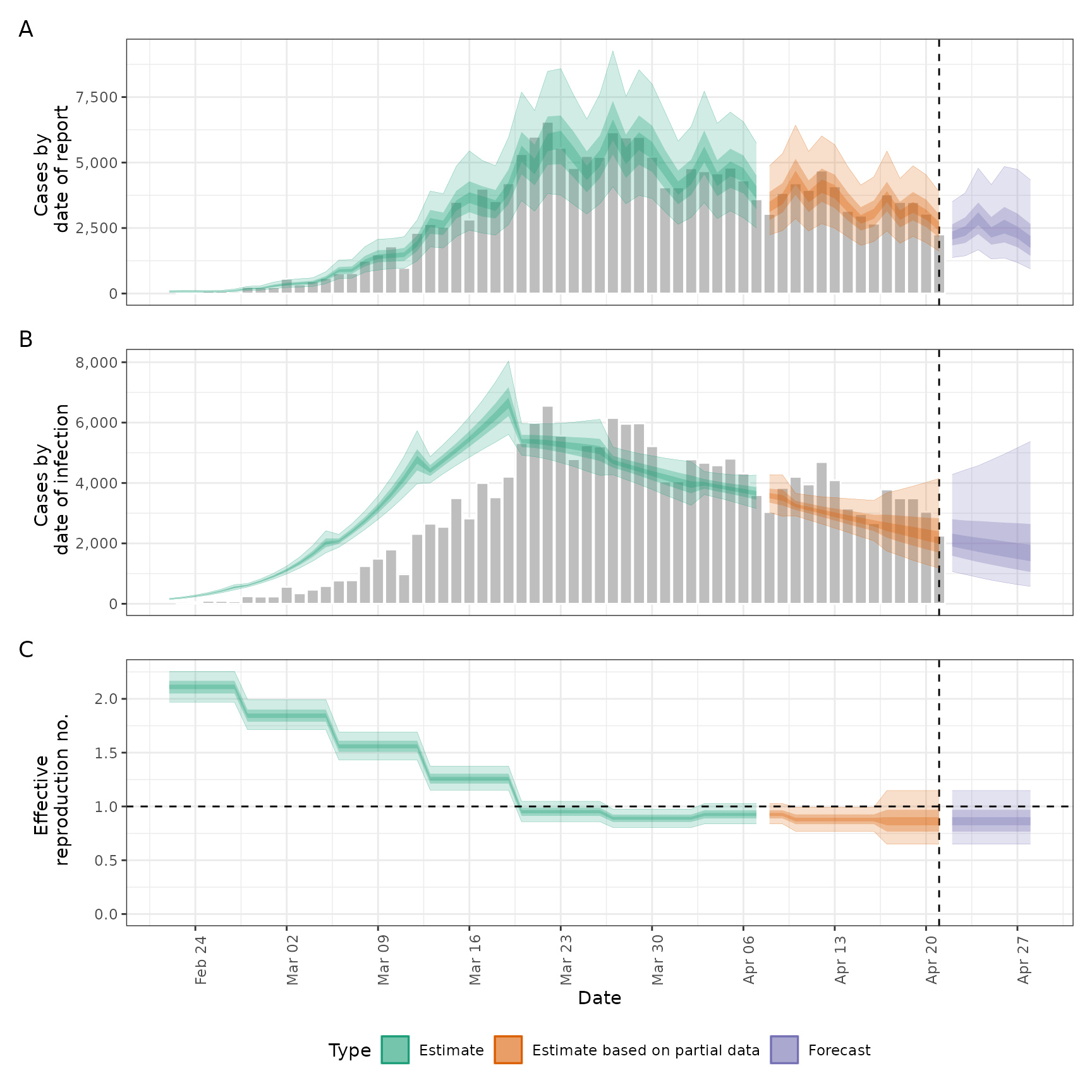# }